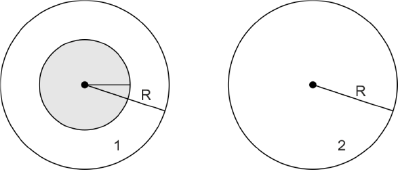Q&A - Ask Doubts and Get Answers

Sort by :
Clear All
Q

In which of the following devices , the eddy current  effect is not used ?

• Option 1)

induction furnace

• Option 2)

magnetic braking in train

• Option 3)

electromagnet

• Option 4)

electric heater

electric heater Option 1)induction furnace Option 2)magnetic braking in train Option 3)electromagnet Option 4)electric heater

A 800 turn coil  of  effective area   is kept perpendicular to a magnetic field  . When the plane of the coil is rotated by 90 around  any of its coplanar axis in 0.1 s , the emf induced in the coil will be :

• Option 1)

2 V

• Option 2)

0.2 V

• Option 3)

0.0002 V

• Option 4)

0.02 V

N = 800       case 1    B and A are parallel  So  case 2 B and A are perpendicular  So  Option 1) 2 V  Option 2) 0.2 V  Option 3) 0.0002 V  Option 4) 0.02 V
Medical
96 Views   |

The magnetic potential energy stored in a certain inductor is 25 mJ, when the current in the inductor is 60 mA. This inductor is of inductance

• Option 1)

1.389 H

• Option 2)

138.88 H

• Option 3)

0.138 H

• Option 4)

13.89 H

As we have learned @8482 Option 1) 1.389 H Option 2) 138.88 H Option 3) 0.138 H Option 4) 13.89 H
Medical
390 Views   |

Which of the following combinations should be selected for better tuning of an L-C-R circuit used for communication?

• Option 1)

R = 20,  L = 1.5 H,  C = 35F

• Option 2)

R = 25,  L = 2.5 H,  C = 45F

• Option 3)

R = 15,  L = 3.5 H,  C = 30F

• Option 4)

R = 25,  L = 1.5 H,  C = 45F

As we learnt in  Q factor - - wherein L - inductance C - capacitance     Quality factor of L-C-R circuit Clearly, Q3  is maximum of Q1 , Q2  and Q4 i.e for Q to be high, L should be high and C should be low.   Option 1) R = 20 ,  L = 1.5 H,  C = 35 F this is the incorrect option Option 2) R = 25 ,  L = 2.5 H,  C = 45 F this is the incorrect option Option 3) R = 15 ,  L = 3.5 H,  C =...
Medical
423 Views   |

The potential differences across the resistance, capacitance and inductance are 80 V, 40 V and 100 V respectively in an L-C-R circuit. The power factor of this circuit is

• Option 1)

0.4

• Option 2)

0.5

• Option 3)

0.8

• Option 4)

1.0

VR = 80 V, VC = 40V, VL = 100V  Power factor,  =  Option 1) 0.4 this is the incorrect option Option 2) 0.5 this is the incorrect option Option 3) 0.8 this is the correct option Option 4) 1.0 this is the incorrect option
Medical
106 Views   |

A 100resistance and a capacitor of 100reactance are connected in series across a 220 V source. When the capacitor is 50% charged, the peak value of the displacement current is

• Option 1)

2.2 A

• Option 2)

11 A

• Option 3)

4.4 A

• Option 4)As we discussed Impedence - -     Peak current - -     Option 1) 2.2 A Option is correct Option 2) 11 A Option is incorrect Option 3) 4.4 A Option is incorrect Option 4) Option is incorrect
Medical
105 Views   |

A uniform magnetic field is restricted within a region of radius r. The magnetic field changes with time at a rate. Loop 1 of radius R > r

encloses the region r and loop 2 of radius R is outside the region of magnetic field as shown in the figure below. Then the e.m.f. generated is• Option 1)

zero in loop 1 and zero in loop 2

• Option 2)in loop 1 andin loop 2

• Option 3)in loop 1 and zero in loop 2

• Option 4)in loop 1 and zero in loop 2

As discussed in Rate of change of magnetic Flux -   - wherein change in flux     In loop 1,   In loop 2,   Option 1) zero in loop 1 and zero in loop 2 This option is incorrect. Option 2) in loop 1 and in loop 2 This option is incorrect. Option 3) in loop 1 and zero in loop 2 This option is incorrect. Option 4) in loop 1 and zero in loop 2 This option is correct.
Exams
Articles
Questions